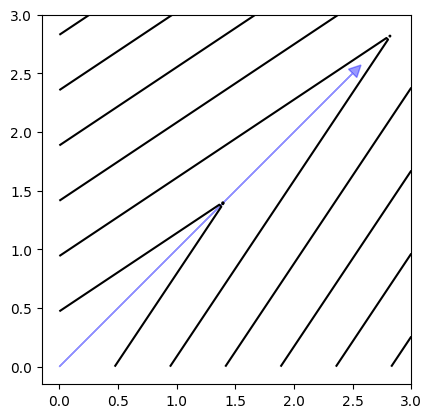Version: 0.5.0

# Decomposition¶

Also, multi-objective problems can be decomposed using a scalarization function. In the following, the contour lines of different methods are shown.

Let us first make the necessary imports and define the points in the design space:

:

import matplotlib.pyplot as plt
import numpy as np
from pymoo.factory import get_decomposition
from pymoo.util.misc import all_combinations

# number of points to be used for plotting
n_points = 100

# the xlim
P = np.linspace(0, 3, n_points)

# used for the meshgrid
X = all_combinations(P,P)


A method to plot the contours:

:

def plot_contour(X, F):
_X = X[:, 0].reshape((n_points,n_points))
_Y = X[:, 1].reshape((n_points,n_points))
_Z = F.reshape((n_points,n_points))

fig, ax = plt.subplots()
ax.contour(_X,_Y, _Z, colors='black')
ax.set_aspect('equal')


And then define the weights to be used by the decomposition functions:

:

weights = [0.5, 0.5]


## Weighted Sum¶

:

plot_contour(X, get_decomposition("weighted-sum").do(X, weights=weights))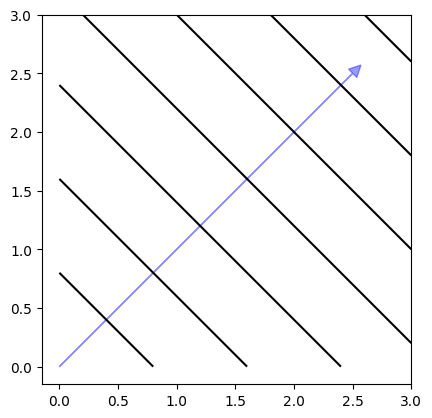## Tchebysheff¶

:

plot_contour(X, get_decomposition("tchebi").do(X, weights=weights))## Achievement Scalarization Function (ASF)¶

Details can be found in .

:

plot_contour(X, get_decomposition("asf", eps=0).do(X, weights=weights))## Augmented Achievement Scalarization Function (AASF)¶

Details can be found in .

:

plot_contour(X, get_decomposition("aasf", eps=0, beta=5).do(X, weights=weights))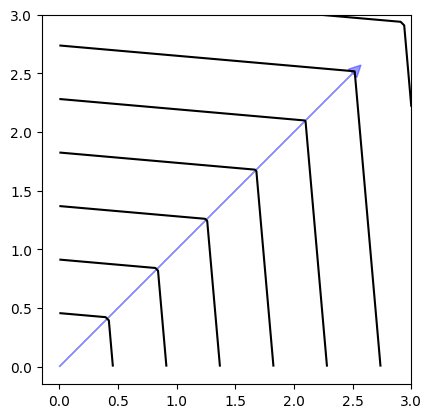:

plot_contour(X, get_decomposition("aasf", eps=0, beta=25).do(X, weights=weights))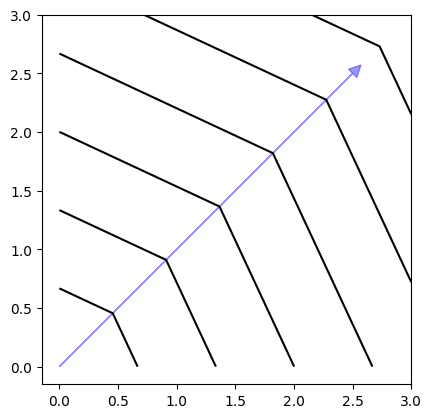## PBI¶

:

plot_contour(X, get_decomposition("pbi", theta=0.5, eps=0).do(X, weights = weights))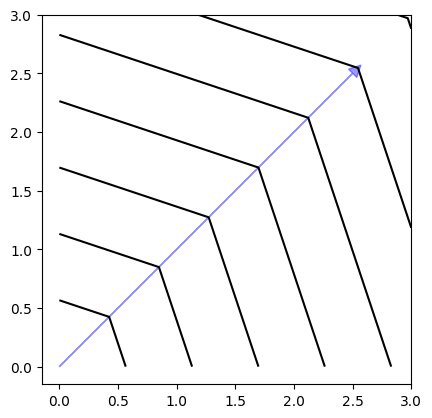:

plot_contour(X, get_decomposition("pbi", theta=1, eps=0).do(X, weights = weights)):

plot_contour(X, get_decomposition("pbi", theta=5, eps=0).do(X, weights = weights))